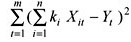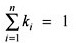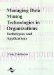# Methodology and Experiments

 < Day Day Up >

This study adopts the approach of using the constrained OLS in estimating the "optimal" weights as has been successfully applied by numerous authors. Due to the convenience of available software, a quadratic programming approach to this problem is adopted. The aim is to choose a method which will yield sample minimum sum of squared forecast errors for the combined forecasts. Basically, given a set of forecasts from methods i = 1 to n over m time periods t = 1 to m, Xit, the problem is to determine the fixed weights ki over the m periods for each forecasting method, i =1 to n, which give the minimum squared forecast errors for the actual data values Yt (t = 1 to m). This can be formulated as a quadratic programming problem with the weights ki as the decision variables as follows:

 Minimizesubject toand k ≥i 0

If a system of fixed weights for monthly data is used, the above model will be run at regular intervals, e.g., once per year. The weights found as solution values are then used as fixed weights month by month for combining the forecasts until a revision is made at the start of the next year. If a "rolling window" is used, then the above model is applied successively in each period minimizing the errors over the preceding m periods, where m is the length in periods of the "rolling window."

 < Day Day Up >Managing Data Mining Technologies in Organizations: Techniques and Applications
ISBN: 1591400570
EAN: 2147483647
Year: 2003
Pages: 174